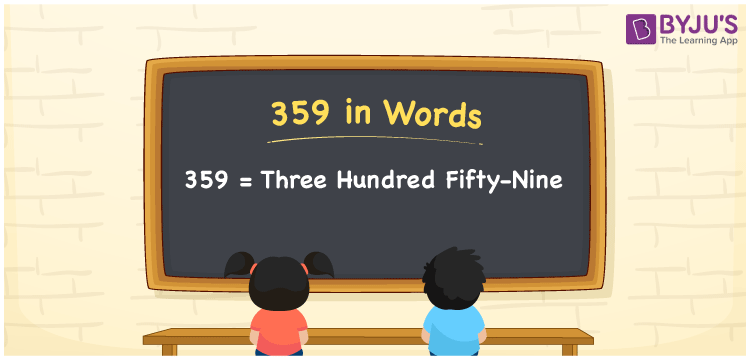# 359 in words

359 in words is written as Three Hundred and Fifty Nine. 359 represents the count or value. The article on Counting Numbers can give you an idea about count or counting. The number 359 is a 3 digit number that is used in expressions related to money, days, distance, length, weight and so on. Let us consider an example for 359. “The height of this rock mountain is Three Hundred and Fifty Nine meters.”

 359 in words Three Hundred and Fifty Nine Three Hundred and Fifty Nine in Numbers 359

## 359 in English Words## How to Write 359 in Words?

We can convert 359 to words using a place value chart. The number 359 has 3 digits, so let’s make a chart that shows the place value up to 3 digits.

 Hundreds Tens Ones 3 5 9

Thus, we can write the expanded form as:

3 × Hundred + 5 × Ten + 9 × One

= 3 × 100 + 5 × 10 + 9 × 1

= 359

= Three Hundred and Fifty Nine.

359 is the natural number that is succeeded by 358 and preceded by 360.

359 in words – Three Hundred and Fifty Nine.

Is 359 an odd number? – Yes.

Is 359 an even number? – No.

Is 359 a perfect square number? – No.

Is 359 a perfect cube number? – No.

Is 359 a prime number? – Yes.

Is 359 a composite number? – No.

## Solved Example

1. Write the number 359 in expanded form

Solution: 3 × 100 + 5 × 10 + 9 × 1

We can write 359 = 300 + 50 + 9

= 3 × 100 + 5 × 10 + 9 × 1.

## Frequently Asked Questions on 359 in words

Q1

### How to write the number 359 in words?

359 in words is written as Three Hundred and Fifty Nine.
Q2

### Is 359 divisible by 3?

No. 359 is not divisible by 3.
Q3

### Is 359 a prime number?

Yes. 359 is a prime number.